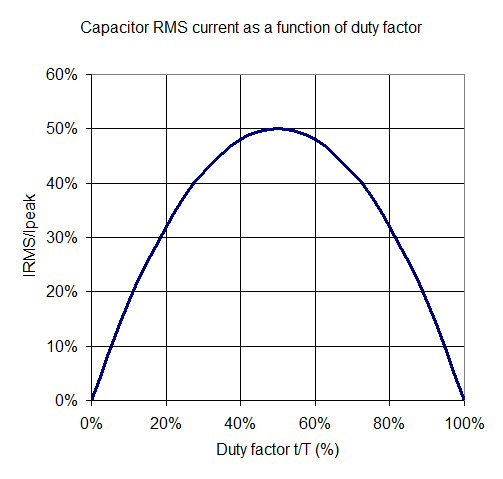# Capacitor ESR Effects

Click here to go to our main page on power conditioning circuits

New for December 2018: here's a new page that provides an updated calculation for charge storage dissipation.  There are problems with the method that is presented on this page.

This page will show you why you need to worry about the equivalent series resistance (ESR) of charge storage capacitors in pulsed applications. In some cases, the number of capacitors you need will be driven by heating, not by voltage droop. ESR is a type of parasitic element, others are discussed here.

In the case of electrolytic capacitors powering a pulsed transmitter, the ESR of a single 4.7 uF capacitor might be on the order of one ohm. The equivalent series resistance of a capacitor can be a strong function of voltage in ceramic capacitors, but a near constant in electrolytics. Often, in pulsed transmitters, you will have to design a modulator circuit that supplies high peak currents to the power amps, with fast rise and fall times. The power supply will be located far enough from the amplifier/modulator so that it can't source the current pulses. This is when charge storage capacitors take over the heavy lifting.

In the chart below we examine a charge storage system (yes, we need to post a schematic too!) where the current from the power supply is effectively decoupled from the charge storage capacitor bank. The caps are located right next to the modulator circuit, which switches the transmitter on for 10 usecs, then off for 40 usecs (20% duty cycle). The power supply supplies direct current of 0.2 amps (peak current x duty factor). The caps supply the other 0.8 amps during the pulse, and charge up at -0.2 amps when the transmitter is off (so that charge in=charge out).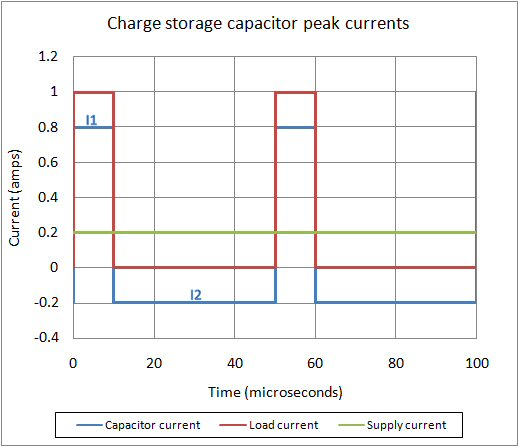The problem with this analysis is as follows: The total inrush and outrush charge (current x tine) to the capacitor has to be equal.  In the plot, you see that is not the case.  Indeed, the capacitor current will not be flat as the cap recharges.  Go here for a better way to analyze this.

### Voltage drop effect

The voltage the the charge storage capacitor bank supplies is reduced by its equivalent series resistance. For the net ESR of the bank you must compute the ESR of all capacitors in parallel. The usual assumption is that they are all the same (but they are not in reality.) But capacitors are usually specified at a maximum ESR so this calculation will be slightly pessimistic and will give your design some margin.

In the above example, if the charge storage presents 1 ohm of ESR, it will contribute one volt drop in the system; thus if you wanted to drain-pulse a GaAs amplifier at 8 volts you would need a 9 volt supply. The next part of this page will explain how the power dissipation due to ESR is calculated.

### Power dissipation effect

Dissipated power across a resistor is equal to the RMS value of the pulsed current waveform squared times the resistance. For N capacitors in parallel, divide the single-cap value of ESR by N.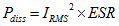Next we need to compute the RMS current of the waveform.

#### The art of calculus without doing calculus...

In order to RMS current, you need to time-average the square of the current, then take the square-root of the result. Most often your microwave instructor will resort to integral signs at this point. But for square current pulses, you can almost do this in your head and don't need to resort to calculus! We will follow Bruce Lee's example:

The art of fighting without fighting from Enter the Dragon

During the current pulse, it the power supply is entirely decoupled from the charge storage, the capacitor source the peak current minus the average current (I1):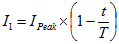When the transmitter is off, the caps recharge at current I2: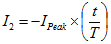Now we merely time-average the square of the current waveform to get RMS current. The equations below shown the calculation step-by-step.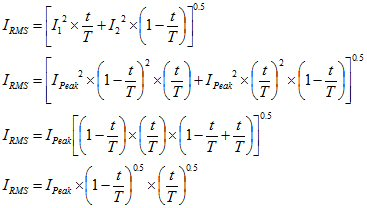With the RMS current now calculated, we can now calculate the dissipation due to ESR: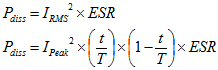Last, let's look at the RMS current as a percentage of peak current. For the transmitter fully on or off (DF=100% or DF=0%), the capacitors supply zero current. The maximum current occurs when DF=50% (a square wave). At 25% or 75% DF, the ratio is 3/16.